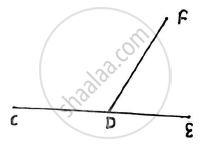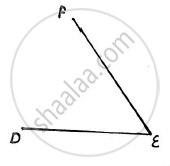# What is a Zero Force Member in a Truss? with Examples State the Conditions for a Zero Force Member. - Engineering Mechanics

Short Note

What is a zero force member in a truss? With examples state the conditions for a zero force member.

#### Solution

1. In engineering mechanics, a zero force member is a member (a single truss segment) in a truss which, given a specific load, is at rest that is it is neither in tension, nor in compression
2.The conditions for a zero force member are :
(a)In a truss,at a joint there are only three members of which two are collinear and if the joint has no external load then the non collinear members is a zero force member.e.g.: DF is a zero force member in the given figure.
(b) In a truss,if at an unsupported joint there are only two members and if the joint has no external load then both the members are zero force members.e.g.:DE and EF are zero force members in the given figure.

Concept: Analysis of Plane Trusses by Using Method of Joints
Is there an error in this question or solution?